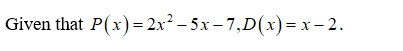# Two polynomials P and D are given. use  either synthetic division or long division to divide P(x) by D(x) and express the quotient in P(x)/D(x) in the form P(x)/D(x)=Q(x)+R(x)/D(x)P(x)=2x^2-5x-7, D(x)=x-2

Question
2 views

Two polynomials P and D are given. use  either synthetic division or long division to divide P(x) by D(x) and express the quotient in P(x)/D(x) in the form P(x)/D(x)=Q(x)+R(x)/D(x)

P(x)=2x^2-5x-7, D(x)=x-2

check_circle

Step 1...

### Want to see the full answer?

See Solution

#### Want to see this answer and more?

Solutions are written by subject experts who are available 24/7. Questions are typically answered within 1 hour.*

See Solution
*Response times may vary by subject and question.
Tagged in